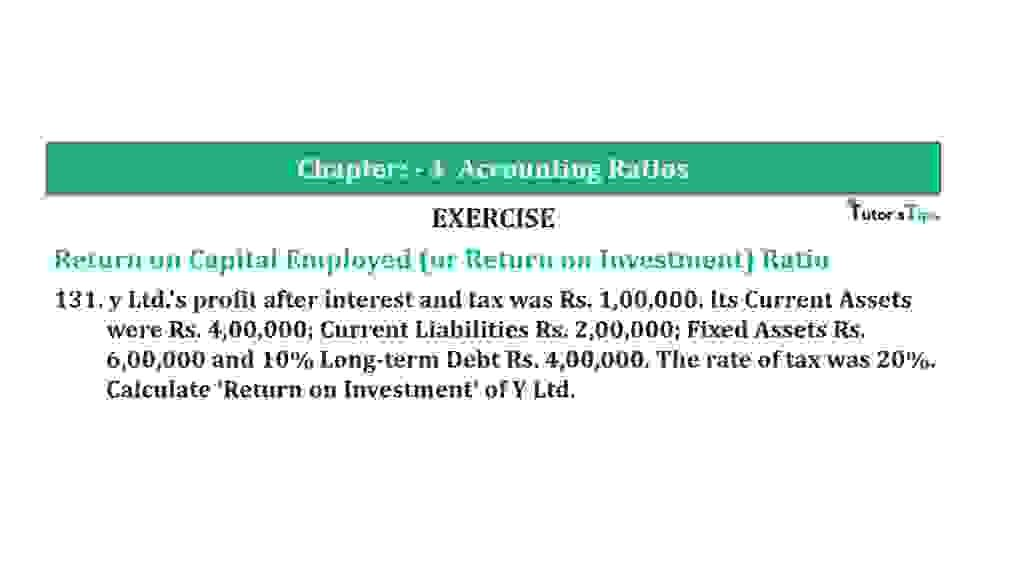# Question 131 Chapter 4 of +2-B – T.S. Grewal 12 ClassQuestion 131 Chapter 4 of +2-B

Return on Capital Employed (or Return on Investment) Ratio

131. y Ltd.’s profit after interest and tax was Rs. 1,00,000. Its Current Assets
were Rs. 4,00,000; Current Liabilities Rs. 2,00,000; Fixed Assets Rs.
6,00,000 and 10% Long-term Debt Rs. 4,00,000. The rate of tax was 20%.
Calculate ‘Return on Investment’ of Y Ltd.

### The solution of Question 131 Chapter 4 of +2-B: –

 Profit before tax = 100 Tax = 20 Profit after tax = 100 – 20 = 80 Profit after tax = Rs. 1,00,000
 Profit before tax = 100 X Rs.1,00,000 80
 = Rs. 1,25,000 Interest on long-term borrowings = Rs. 4,00, 000 ×10% = Rs. 40,000 Capital Employed = Fixed Assets+ Current Assets – Current Liabilities = Rs. 6,00,000 + Rs. 4,00,000 – Rs. 2,00,000 = Rs. 8,00,000

 Net Profit Ratio = Profit before Interest and Tax X 100 Capital Employed
 Net Profit Ratio = Rs. 1,65,000 X 100 Rs. 8,00,000 = 20.63%

Balance Sheet: Meaning, Format & Examples

Thanks, Please Like and share with your friends

Comment if you have any question.

Also, Check out the solved question of previous Chapters: –

### T.S. Grewal’s Double Entry Book Keeping (Vol. II: Accounting for Companies)T.S. Grewal’s Analysis of Financial Statements